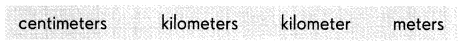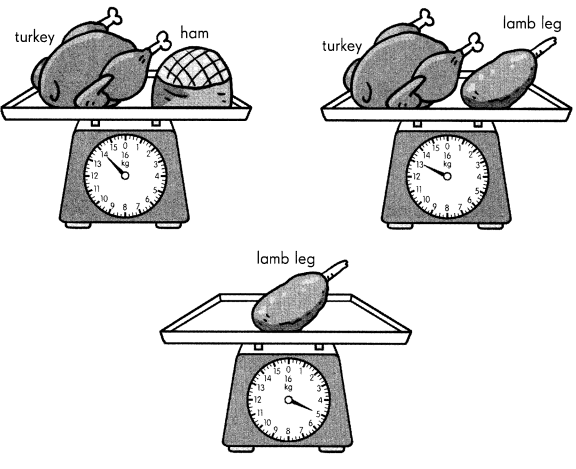# Math in Focus Grade 3 Chapter 11 Answer Key Metric Length, Mass, and Volume

Practice the problems of Math in Focus Grade 3 Workbook Answer Key Chapter 11 Metric Length, Mass, and Volume to score better marks in the exam.

## Math in Focus Grade 3 Chapter 11 Answer Key Metric Length, Mass, and Volume

Challenging Practice

Fill in the correct units of measurement.Question 1.
William and Burt had a race on the running track. William took ten minutes to run one __________, while Burt took 4 minutes to run seven hundred _________.
kilometer and meter
Explanation:
William and Burt had a race on the running track. William took ten minutes to run one kilometer , while Burt took 4 minutes to run seven hundred meters.

Circle the better measurement.

Question 2.
The height of a five-story building is 30 ( meters/centimeters ).
The height of a five-story building is 30 meters
Explanation:
The height of the building cannot be in centimeters
Measure of centimeter is very small

Question 3.
The length of a desk is 1 ( meter/kilometer ).
The length of a desk is 1 meter
Explanation:
The length of the desk cannot be in kilometers
Measure of kilometer is very big

Question 4.
The weight of a large log from a tree is 1 8 ( kilograms/grams ).
The weight of a large log from a tree is 1 8 kilograms
Explanation:
The weight of a large log cannot be in grams
grams is very light weight

Question 5.
The weight of a pen is 1 0 ( grams/kilograms ).
The weight of a pen is 1 0 grams
Explanation:
The weight of a pen cannot be in kilograms
Kilograms will be very heavy

Question 6.
The capacity of a barrel is about 100 ( liters/milliliters ) of water.
The capacity of a barrel is about 100 liters
Explanation:
The capacity of a barrel will be more
but milliliters will be less in mass

Problem Solving

Find the mass of each item.Question 7.
The mass of the lamb leg is _________ kilograms.
The mass of the lamb leg is 5 kilograms.
Explanation:
According to weigh machine the meter shows as the lamb leg weighs 5 kgs

Question 8.
The mass of the turkey is __________ kilograms.